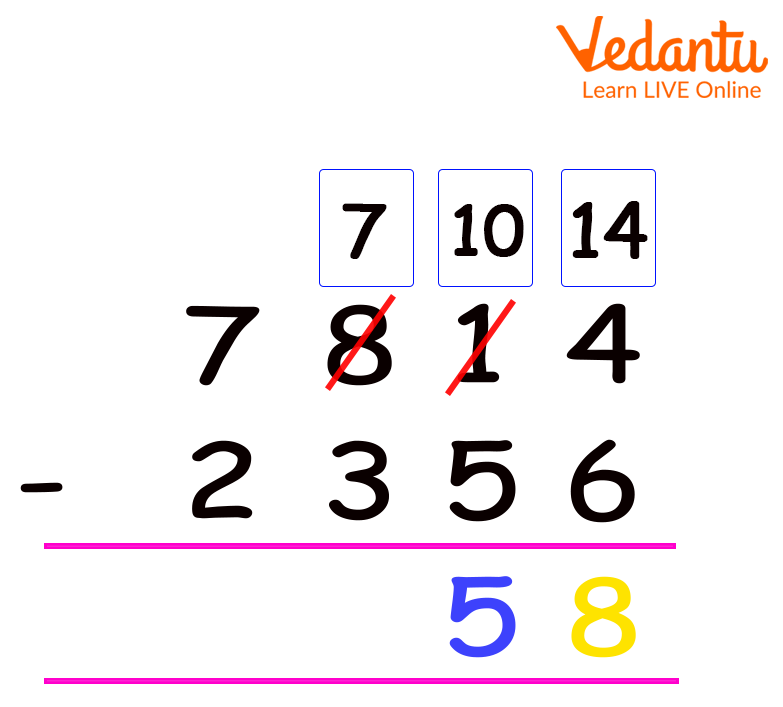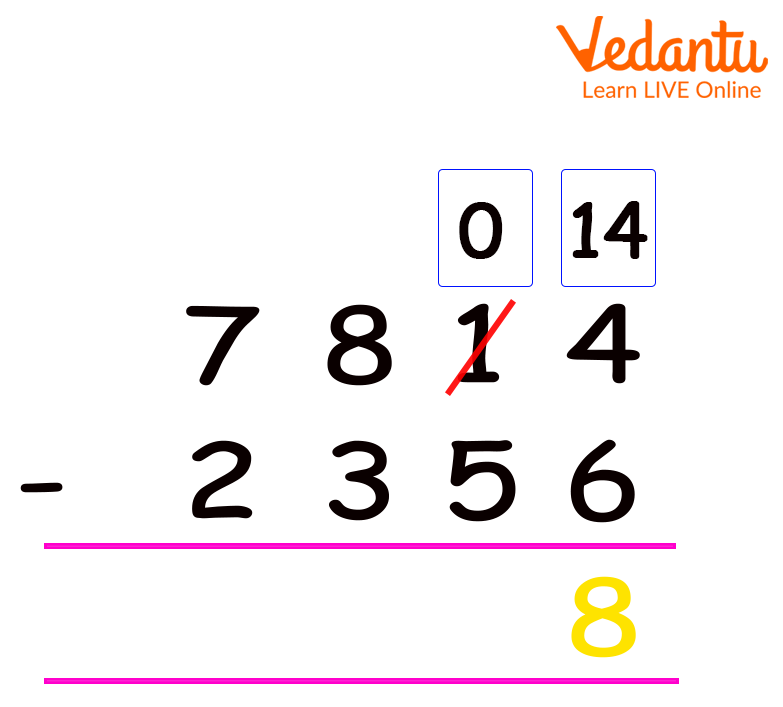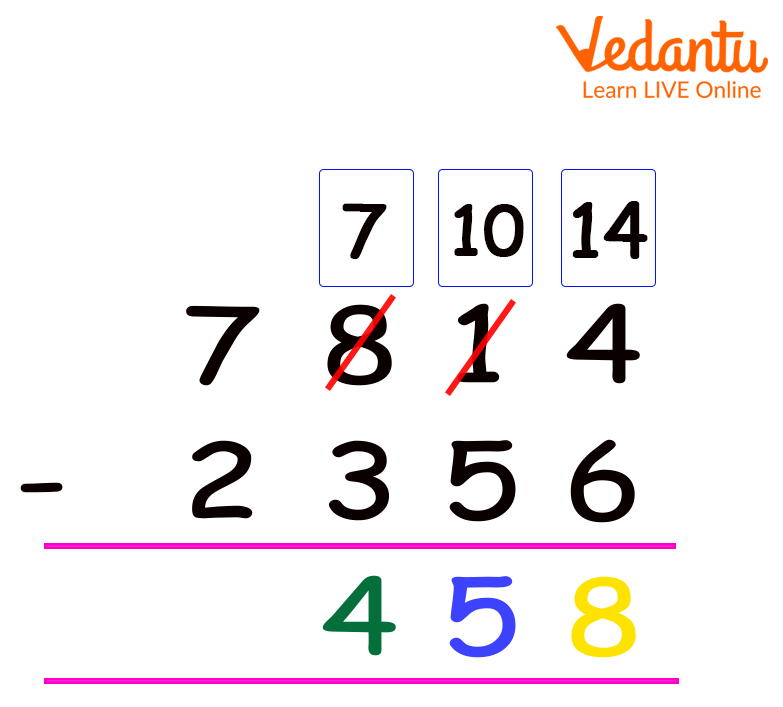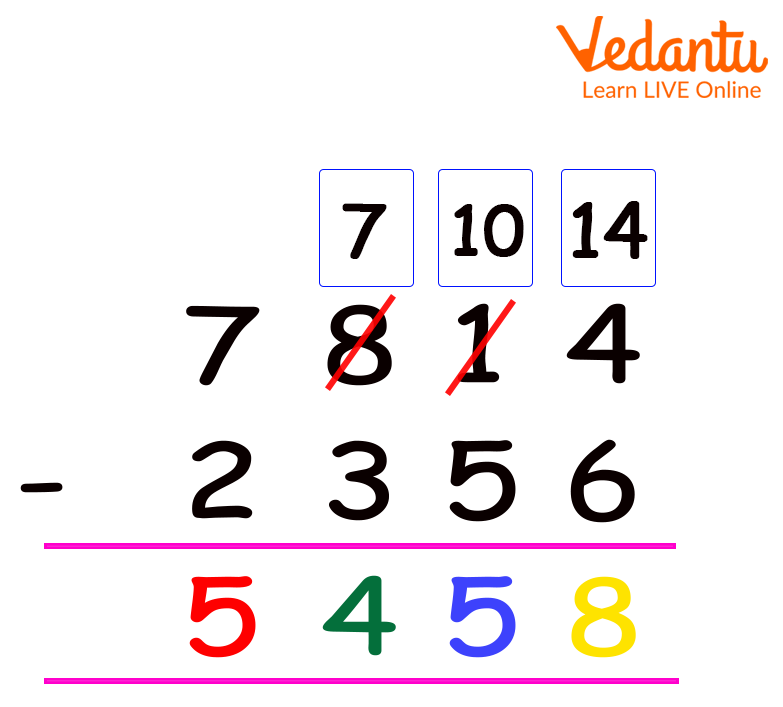Courses
Courses for Kids
Free study material
Free LIVE classes
More

# Subtraction with Regrouping## What is Subtraction?

The concept of the difference in maths is finding the difference between the numbers. Here, the number we are subtracting is subtrahend, the number from which we are subtracting is minuend, and the result we are obtaining is the difference.

The subtraction can be between two one-digit numbers, two-digit numbers, three-digit numbers, four-digit numbers, and so on.

Now, let us understand what subtraction with regrouping is. Well, this concept says that the subtraction process is done by exchanging one ten’s into ten one’s.

Here, we will use the concept of subtraction with regrouping for three to four-digit numbers.

## How to do Subtraction with Regrouping?

Suppose we wish to find the difference between 785 and 468. Both are three-digit numbers, therefore placing one number below the other in order of their place values, we get –Subtraction of two three-digit numbers

In the above case, we conclude that each digit of the subtrahend was less than the given digit in the minuend. So, how do we subtract a subtrahend that is greater at a certain place value than the corresponding value of the minuend? Well, for this, we will understand it using an example.

Now, here if we desire to determine the difference between 2356 and 7814, we will first compare the digits of both the subtrahend and the minuend.

Secondly, we notice that the value of subtrahend is greater than the value of the minuend at a few places.

A question arises: how do we subtract 6 from 4? Here, we use the concept of taking away a digit from the next place value or borrowing a value in such cases. By borrowing, we mean that we borrow 1 from the next number in the place value, like one’s digit taking ‘1’ from the ten’s place value. This is known as regrouping.

Let us solve this step by step.

Step 1: Firstly, subtract 6 from 4. Since we cannot subtract larger numbers from smaller ones, we borrow 1 from the digit that is at the ten’s place in the minuend to make the one’s digit larger.

Like here, in this case, the number is 1, so we borrow 1 from 1, and the 1 at the ten’s place in the minuend turns to 0.

Therefore, the number at the one’s place of the minuend, after borrowing 1 becomes 14. Now, it is easier for us to subtract 6 from 14, and hence, we get 8 at the one’s place as the answer.Step 1 of subtraction with regrouping

Step 2: In the next step, we move to subtract the digits at the ten’s place. Please note that the digit at the ten’s place is now 0, instead of the 1 that we had earlier.

Now, what we do is, we have to subtract 5 from 0, which again is impossible.

However, we repeat the steps again that we did for subtracting the digits at the one’s place.

Now, here, we will borrow 1 from the digit at the hundred’s place, and give it to 0 at the ten’s place. So, now ‘8’ at the hundred’s place of the minuend turns to 7 and the 0 at the ten’s place of the minuend becomes 10. Now, it is easier for us to subtract 5 from 10 and hence we get 5 as the answer at the ten’s place.Step 2 of subtraction with regrouping

Step 3: Now, let us have a look at the values at the hundred’s place. We can see ‘3’ as the subtrahend and 7 as the minuend, which can be subtracted now. Therefore, we get 4 as the answer at the hundred’s place.Step 3 of subtraction with regrouping

Step 4: Lastly, we go next to values at the thousand’s place. Here, we find 2 as the subtrahend and 7 as the minuend. Hence, easily we get 5 as the answer at the thousand’s place.Step 4 of subtraction with regrouping

Hence, using the formula Minuend - Subtrahend = Difference, we get,

7814 – 2356 = 5458 as the answer.

So, this is how we do subtraction with regrouping for two three-digit and two four-digit numbers by using the concept of borrowing.

Last updated date: 02nd Oct 2023
Total views: 139.8k
Views today: 1.39k

## FAQs on Subtraction with Regrouping

1. What is an example of regrouping?

Regrouping in maths is making groups of ten while performing operations like addition or subtraction. For example, in 2 digit addition, we take 15 + 17. In this case, we need to regroup, like firstly, add 5 + 7 to get 12, then 1 + 1 becomes 2 Here, 1 is carried over from 12 in the one’s place and it gets added to ‘2’ at the ten’s place.

Hence, we get (2 + 1) 2 = 32 as our answer.

2. What is the difference between subtraction with regrouping and subtraction without regrouping?

Let us understand this with an example:

Assume that 94 - 66 requires regrouping because four is smaller than six. Subtraction without regrouping says that the number in a place value group of the number we are subtracting from (minuend) is greater than the number in a place value group of the number you're subtracting (subtrahend). So, in the case of subtraction without grouping, the example turns to 94 - 62.